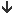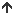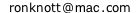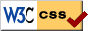# Fractions – Decimals Calculator version 2.6

The Calculator on this page will convert a proper fraction to and from a decimal or radix fraction.
For a more complete Introduction to Fractions and their several kinds of Decimals,
see the related Fractions and Decimals page.

The calculator on this page requires JavaScript but you appear to have switched JavaScript off (it is disabled). If you want to use this calculator please go to the Preferences for this browser and enable JavaScript then Reload this page.
All proper fractions are input as two decimal numbers, the numerator and the denominator. The decimal fractions are shown to any number of decimal places.
An option is to see the "decimal" fraction in another base apart from decimal (base 10) e.g. base 2 (binary), base 8 (octal), base 16 (hexadecimal) or any other base from 2 upwards.
The default base is 10 for decimals, but you can change it to any other value from 2 upwards. All proper fractions are input as decimal numbers. It is only the radix fractions that are given in the specified base.
A decimal fraction in any base other than 10 is called a radix fraction here. For example, 0.1012 is the base-2 (binary) radix fraction form of the proper fraction 5/8 and similarly 0.478 is the base-8 (octal) radix fraction for 39/64.
The "digits" of numbers in a base bigger than 10 are each shown as a separate decimal number with each column (place) separated by a space. For example:
 11/12 + 1/122 in base 12 is shown as 0 . 11 1 1/12 + 1/122 in base 12 is shown as 0 . 1 1 11/122 in base 12 is shown as 0 . 0 11

## to any number of decimal places

The decimal or radix equivalents of fractions here can be given to any number of decimal(radix) places, unlike an ordinary calculator which often only gives up to 12 or 15 significant figures.
All the initial digits are shown but since recurring parts can be very long, you can specify how many of the beginning and ending digits of the period (the outer digits) you want to be shown in the RESULTS. Leaving it empty or entering 0 will give only the number of digits in its fixed and recurring parts and not show any of the decimal/radix places.
In every base, a radix ("decimal") fractional value will only occur in one of the following 3 forms:
terminating:
a finite number of places such as 1/2 = 0.5 and 3/8 = 0.325
purely recurring:
a cycle of digits which repeats for ever, such as 1/3 = 0.33333.... and 4/33 = 0.121212121212.... which are output by the Calculator as 0. and 0. respectively
an initial fixed and finite part followed by an recurring part (the period) which repeats for ever.
such as 52/225 = 0.23111111111... = 0.23 and 1/700 = 0.00 142857 142857 ... = 0.00

The first part after the radix point is the "non-repeating" or fixed part, if any. Leave this empty if your decimal is purely recurring.
The recurring part is always shown here inside square brackets. Leave it empty for a terminating decimal.

### Fraction to Decimal Examples

What is 7/15 as a decimal fraction?
• Enter 7 and 15 as the fraction 7/15
• Alter the number in the Show outer recurring box to the number of decimal places you want displayed.
• click onto "move" the fraction down to the decimals area.
• The fixed and recurring parts show the result
1 initial place, 1 recurring place
0.
[ ]
and the results are also recorded in the RESULTS area:
• 7/15 has 1 initial digit followed by a period of 1 digit
7/15 = 0.4
7/15 = 0.4666666666 ...
What are the first 100 decimals places for the fraction 1/9899?
• Enter the fraction / in the fraction input boxes.
• Enter 100 in the number of outer recurring places box:
show outer recurring .
• Pressbutton:
• 1/9899 is purely recurring with a period of 468 digits
1/9899 = 0.[0001010203050813213455904636832003232649762602283058894837862410344479240327305788463481159713102333...
9575714718658450348520052530558642287099707041115264168097787655318719062531568845337912920497019901]
Does the fraction 68/175 terminate or recurr?
• Enter / in the fraction input boxes
• Enter 0 in the Show outer recurring places box or else leave it empty.
• Press• 68/175 has 2 initial digits followed by a period of 6 digits.
• If you want to see the decimal places, change the number of outer places to 10 or some other value and pressagain.
• 68/175 = 0.38
68/175 = 0.3885714285 ...
• which means the radix fraction begins with 38 followed by 857142 857142 857142 ..., the recurring part being shown in square brackets.
What is 1/3 in binary?
• Change the base to 2:
in base
• In the top part enter 1/3
• Click on• 1/3 = 12/112. In base 2 it is purely recurring with a period of 2 digits
1/3 = 0. in base 2
1/3 = 0.0101010101 ... in base 2

### Decimal to Fraction

The part before any recurring digits (if any) is called the Fixed part of the decimal fraction and the repeating part (if any) is put inside square brackets [ and ]
0 . [ ]
What fraction corresponds to a given decimal fraction, for example 0.0625?
• In the lower part enter 0625 in the Fixed part, leaving the Recurring part empty:
0 . [ ]
• Click on• 0.0625 = 1/16
What is 0.222222.... as an exact fraction?
• Leave the initial Fixed part blank and type 2 in the Recurring part input box:
0 . [ ]
• Press• 0. = 2/9
What is 0.011 in binary (base 2) as a proper fraction?
• Enter 011 as the fixed part and make sure the Recurring part is empty:
0 . [ ]
Change the base to 2:
in base
to indicate the fixed and recurring parts are in binary.
• Click on• 0.011 in base 2 = 3/8
What is the binary (base 2) recurring fraction 0.1001100110011 .... as a proper fraction?
• Enter 1 as the fixed part and 0011 in the Recurring part and change the base to 2
• Click on• 0.1 in base 2 = 3/5
• The following are equally valid inputs for this question:
0 . [ ]
0 . [ ]
What is the fraction proper fraction corresponding to the base 12 fraction 0 . 10 2 11 11 11 ...?
For bases above 10, enter the "digits" as decimal numbers, one for each place, separated by a space.
• Here we enter 0 . [ ]
• Change the base:
in base
• Press thebutton
• 0 . 10 2  in base 12 = 41/48
• The fraction has been entered into the top part so pressto check it, if you like:
• 41/48 = 3 512/4 012. In base 12 it terminates after 2 digits 41/48 = 0 . 10 3 in base 12
• Why is this not the same as the values we gave it: 0 . 10 2 11 11 11 .....?
Because just as 0.19999999.... in decimal is the same as 0.2, so 0.10 2 11 11 11 11 in base 12 is really just 0.10 3!

### Special decimal fractions made from interesting series of numbers

The button lets you select from a choice of series and will fill in a fraction in the top part of the Calculator whose decimal fraction is just that particular series of numbers: e.g. the decimal which is the pattern 0,1,2,3,4,... , with 2 digits (or 1 digit or 3 digits) for each term is 0·00 01 02 03 04 05 06 07 08 09 10 11 ... and, surprisingly, it is the decimal expansion of the fraction 1/9801. Since all fractions terminate or recurr, then eventually the pattern appears to break down.

C A L C U L A T O R
 0 · [ ] in base , show outer recurring show periods as 123 : or  :

R E S U L T S

• 2014 March 24: version 2.6 The Fractions and Decimals introductory page is now back online.
• 2013 April 22: version 2.5
The proper fraction is now converted into the base.
• 2013 March 25: version 2.4
The conversions now fill in the boxes with the converted values.
The fixed digits of a fraction are now always shown in full. However, because recurring parts can be many millions of places long, only a number of "outer" digits - the first few and the last few - of the recurring part of long periods are shown.
You can change the number of "outer" digits (the first few and the last few of the repeating part) to be displayed from 20 to any other value.
• 2013 March 17: version 2.3 simpler layout with arrow buttons to display better on smart phones or tablets.
• 2013 March 16: version 2.2 all input fields can now evaluate an expression using numbers + - * and /
removed bug when converting some fractions to decimals in some bases other than 10; new colour scheme;
• 2013 March 7: version 2.1 now correctly handles fractions bigger than 1.
• 2013 March 5: version 2 includes bases other than 10, new layout.
• 2006 June 2: first version

© 2006-2014 Dr Ron Knott     updated: 10 September 2014